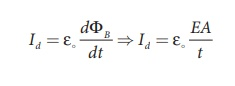Home | | Physics 12th Std | Electromagnetic Waves: Exercises and Example Solved Numerical problems

# Electromagnetic Waves: Exercises and Example Solved Numerical problems

Physics : Electromagnetic Waves: Solved Example Problems with Answers, Solution and Book back Exercises Problems with Answers

## Displacement current and MaxwellŌĆÖs correction to Ampere's circuital law: Solved Example Problems

### EXAMPLE 5.1

Consider a parallel plate capacitor which is maintained at potential of 200 V. If the separation distance between the plates of the capacitor and area of the plates are 1 and 20 cm2. Calculate the displacement current for the time in ┬Ąs.

### Solution

Potential difference between the plates of the capacitor, V = 200 V

The distance between the plates,

d = 1 mm = 1 ├Ś 10-3 m

Area of the plates of the capacitor,

A = 20 cm2 = 20 ├Ś 10-4 m2

Time is given in micro-second, ┬Ąs = 10-6 s

Displacement currentBut electric field, E = V/d

Therefore,= 35400 ├Ś10ŌłÆ7 = 3 .5 mA

## Refractive index of the medium: Solved Example Problems

EXAMPLE 5.2

The relative magnetic permeability of the medium is 2.5 and the relative electrical permittivity of the medium is 2.25. Compute the refractive index of the medium.

Solution

Dielectric constant (relative permeability of the medium) is ╬Ąr = 2.25

Magnetic permeability is ┬Ąr = 2.5

Refractive index of the medium,

n = ŌłÜ ╬Ąr┬Ąr  = ŌłÜ [2 . 25 ├Ś 2.5] = 2.37

## Sources of electromagnetic Waves: Solved Example Problems

EXAMPLE 5.3

Compute the speed of the electromagnetic wave in a medium if the amplitude of electric and magnetic fields are 3 ├Ś 104 N C-1 and 2 ├Ś 10-4 T, respectively.

Solution

The amplitude of the electric field, Eo = 3 ├Ś 104 N C-1

The amplitude of the magnetic field, Bo = 2 ├Ś 10-4 T. Therefore, speed of the electromagnetic wave in a medium is

= 3 ├Ś104 / 2 ├Ś10ŌłÆ4 = 1.5├Ś108 msŌłÆ1

## Electromagnetic spectrum: Solved Example Problems

EXAMPLE 5.4

A magnetron in a microwave oven emits electromagnetic waves (em waves) with frequency f = 2450 MHz. What magnetic field strength is required for electrons to move in circular paths with this frequency?.

Solution

Frequency of the electromagnetic waves given is f = 2450 MHz

The corresponding angular frequency is

Žē= 2ŽĆf = 2 x 3.14 x 2450 x 106

= 15,386 x 106 Hz

= 1.54 ├Ś 1010 s-1

The magnetic field B = meŽē / |q|

Mass of the electron, me = 9.22 x 10-31 kg

Charge of the electron

q =ŌłÆ1 . 60 ├Ś10ŌłÆ 19 C ŌćÆ |q| = 1.60 ├Ś10ŌłÆ19 CB = 0.0887 T

This magnetic field can be easily produced with a permanent magnet. So, electromagnetic waves of frequency 2450 MHz can be used for heating and cooking food because they are strongly absorbed by water molecules.

Tags : Physics , 12th Physics : Electromagnetic Waves
Study Material, Lecturing Notes, Assignment, Reference, Wiki description explanation, brief detail
12th Physics : Electromagnetic Waves : Electromagnetic Waves: Exercises and Example Solved Numerical problems | Physics